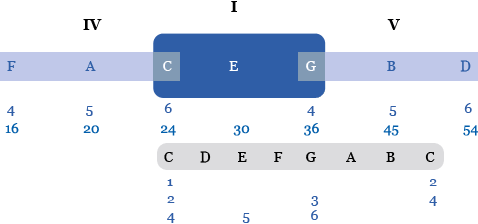Home Basic Concepts Properties of sound Tuning systems Supplementary material
Suomeksi | In English

# Just intonation

The diatonic scale can also be formed using the tones of a harmonic series. A pure fifth can be found between the 2nd and the 3rd harmonic (a ratio of 2:3 or 3:2, depending on which harmonic is mentioned first), while a pure fourth can be found between the 3rd and the 4th harmonic (a ratio of 4:3). The resulting fourth and fifth tone of the major scale (fa and so, see diagram) are identical to the fourth and fifth tone of the Pythagorean scale. The third tone of the scale, the major third, however, deviates from the Pythagorean tuning considerably. The ratio of the Pythagorean third is (3/2)4, or 81/65 (approximately 1.2656...), while the pure third would be 5:4 (1.25).

There are several ways to express the relationships between different tuning systems. The following diagram uses integers instead of ratios. The pitch names (C, D, E...) have been replaced by the relative solféges, because both the just intonation and the Pythagorean tuning are key specific. The octave ratio remains the same: the low do is 24, while the high do is 48. Only integer values are used in a just tempering, as the system is based on simple numeric ratios. In Pythagorean tuning, more decimals start to appear as one proceeds along the series of fifths (FA-DO-SO-RE...); the previous tone position is always at a ratio of 2/3 or 4/3 to the next tone position. For comparison, the bottom line features the approximate values in an equal temperament.

 DO RE MI FA SO LA TI DO Just 24 27 30 32 36 40 45 48 Pythagorean 24 27 30,375 32 36 40,5 45,5625 48 Equal 24 26,939 30,238 32,036 35,959 40,363 45,306 48

Each tone of the chord can be assigned a numeric value that results in simple mathematical proportions:The diagram is an adaptation of Donkin's 1870 artificial scale. The proportions between the tones of the major tritone are 4:5:6  directly from the harmonic series.

The successive tones of the longer numeric series have simple numeric proportions, but A (20) and D (54) do not. By transferring A to the same octave range with D (i.e. by multiplying the number by four), a proportion of 54:80 is established for the fifth. The difference to the pure fifth is now the syntonic comma (a ratio of 81:80, or 21.5 C), because 54:81 = 2:3 (both numbers are divisible by 27).

The following notation example illustrates the differences between the tuning levels of the various scales by placing the highest tones on the right. The pitch of the third tone, for example, is very different in each of the scales mentioned. The bottom set of lines features a uniform equal-tempered scale.